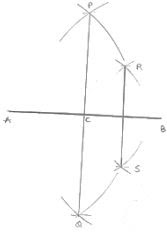# Chapter 17 Constructions RD Sharma Solutions Exercise 17.1 Class 9 Maths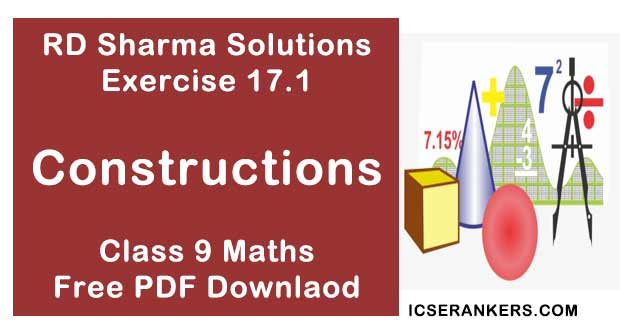Chapter Name RD Sharma Chapter 17 Constructions Exercise 17.1 Book Name RD Sharma Mathematics for Class 10 Other Exercises Exercise 17.2Exercise 17.3 Related Study NCERT Solutions for Class 10 Maths

### Exercise 17.1 Solutions

1. Draw a line segment of length 8.6 cm. Bisect it and measure the length of each part.

Solution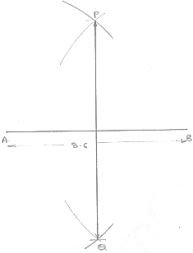Steps of construction :
1. Draw a line segment AB of 8.6 cm
2. With center A and radius more than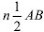, draw arcs, one on each side of AB
3. With center B and same radius, draw arcs cutting the previous arcs at P and Q respectively
4. Join PQ
∴ AC = BC = 4.3 cm

2. Draw a line segment AB of length 5.8 cm. Draw the perpendicular bisector of this line segment.
Solution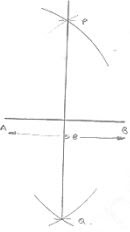Steps of construction :
1. Draw a line segment AB of 5.8 cm
2. With center A and radius more than 1/2 AB, draw arcs with one on each side of AB
3. With center B and same radius draw arcs cutting the previous arcs at P and Q respectively.
4. Join PQ
Hence, PQ is the perpendicular bisector of AB.

3. Draw a circle with centre at point O and radius 5 cm. Draw its chord AB, draw the perpendicular bisector of line segment AB. Does it pass through the centre of the circle ?
Solution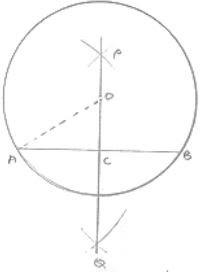Steps of construction :
1. With center O and radius 5cm draw a circle
2. Draw a chord AB.
3. With center A and radius more than 1/2 AB, draw arcs one on each side of
4. With center B and same radius draw arcs cutting previous arcs at P and Q respectively.
5. Join PQ
∴ Yes perpendicular bisector PQ of AB passes through center of the circle.

4. Draw a circle with centre at point O. Draw its two chords AB and CD such that AB is not
parallel to CD. Draw the perpendicular bisectors of AB and CD. At what point do they
intersect?
Solution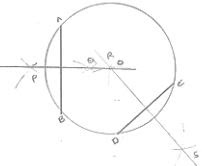Steps of construction :
1. With center O and any radius, draw a circle
2. Draw two chords AB and CD.
3. With center A and radius more than 1/2 AB, draw arcs, one on each side of AB
4. With center B and same radius draw arcs cutting previous arcs at P and Q respectively.
5. Join PQ
6. With center D and radius more than 1/2 DC. draw arcs, one on each side of DC
7. With center C and same radius, draw arcs cutting previous arcs at R and S respectively
8. Join RS
Both perpendicular bisector PQ and RS intersect each other at the center O of the circle.

5. Draw a line segment of length 10cm and bisect it. Further bisect one of the equal parts and measure its length.
Solution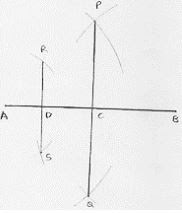Steps of construction :
1. Draw line segment AB of 10 cm
2. With center A and radius more than 1/2AB, draw arcs once on each side of AB
3. With center B and same radius draw arcs cutting previous arcs at P and Q respectively.
4. Join PQ and which intersect AB at C
5. With center A and radius more than 1/2 AC, drawing on each side of AC.
6. With center C and same radius, draw arcs cutting previous arcs at R and S respectively.
7. Join RS and which intersect AC at b.

6. Draw a line segment AB and bisect it. Bisect one of the equal parts to obtain a line segment of length 1/2 (AB).
Solution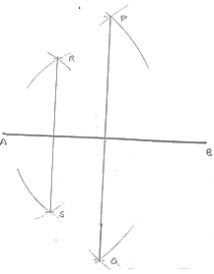Steps of construction :
1. Draw a line segment AB
2. With center A and radius more than 1/2 AB, draw arcs one on each side of AB
3. With center B and same radius draw arcs cutting previous arcs at P and Q respectively.
4. Join PQ and which intersect AB at C.
5. With center A and radius more than 1/2 AC, draw arcs, one on each side of AC.
6. With center C and same radius, draw arcs cutting previous arcs at R and S respectively.
7. Join RS and which intersect AC at D